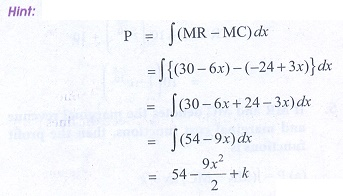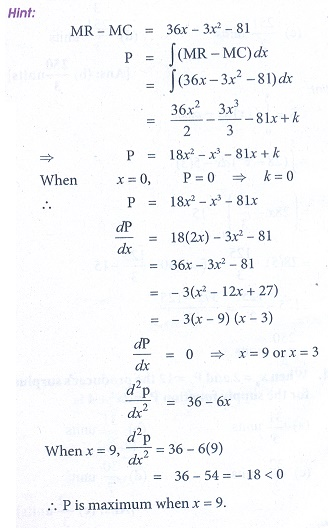Home | | Business Maths 12th Std | Choose the best answer form the given alternatives

# Choose the best answer form the given alternatives

Maths: Integral Calculus: Multiple choice questions with Answers, Solution and Explanation - Book back 1 mark questions and answers with solution for Exercise Problems

Choose the best answer form the given alternatives

1. Area bounded by the curve y = x ( 4 ŌłÆ x) between the limits 0 and 4 with x ŌłÆ axis is

(a) 30/0 sq.units

(b) 31/2 sq.units

(c) 32/3 sq.units

(d) 15/2 sq.units2. Area bounded by the curve y = eŌłÆ2x between the limits 0 Ōēż x Ōēż Ōł× is

(a) 1 sq.units

(b) 1/2 sq.unit

(c) 5 sq.units

(d) 2 sq.units3. Area bounded by the curve y =1/x between the limits 1 and 2 is

(a) log2 sq.units x

(c) log3 sq.units

(d) log 4 sq.units

(b) log5 sq.units4. If the marginal revenue function of a firm is MR= e ŌĆōx/10 , then revenue is

(a) ŌłÆ10e-x/10

(b) 1 ŌłÆ e-x/10

(c)10 (1 ŌłÆ e-x/10)

(d) e-x/10 + 105. If MR and MC denotes the marginal revenue and marginal cost functions, then the profit functions is

(a) P = Ōł½ ( MR ŌłÆ MC ) dx + k

(b) P = Ōł½ ( MR + MC ) dx + k

(c) P = Ōł½ ( MR )( MC )dx + k

(d) P = Ōł½ ( R ŌĆō C) dx + k6. The demand and supply functions are given by D ( x)= 16 ŌłÆ x2 and S ( x) = 2x2 + 4 are under perfect competition, then the equilibrium price x is

(a) 2

(b) 3

(c) 4

(d) 57. The marginal revenue and marginal cost functions of a company are MR = 30 ŌłÆ 6x and MC = ŌłÆ24 + 3x where x is the product, then the profit function is

(a) 9x2 + 54x

(b) 9x2 ŌłÆ 54x

(c) 54x ŌłÆ 9x2/2

(d) 54x ŌĆō [9x2/2] + k8. The given demand and supply function are given by D ( x) = 20 ŌłÆ 5x and S ( x) = 4x + 8 if they are under perfect competition then the equilibrium demand is

(a) 40

(b) 41/2

(c) 40/3

(d) 41/59. If the marginal revenue MR = 35 + 7x ŌłÆ 3x2 , then the average revenue AR is

(a) 35x + 7x2/2 ŌłÆ x3

(b) 35 + 7x/2 ŌłÆ x2

(c) 35 + 7x/2  + x2

(d) 35 + 7x + x210. The profit of a function p(x) is maximum when

(a) MC ŌłÆ MR = 0

(b) MC=0

(c) MR=0

(d) MC+MR=011. For the demand function p(x), the elasticity of demand with respect to price is unity then

(a) revenue is constant

(b) cost function is constant

(c) profit is constant

(d) none of these12. The demand function for the marginal function MR = 100 ŌłÆ 9x2 is

(a) 100 ŌłÆ 3x2

(b)100x ŌłÆ 3x2

(c)100x ŌłÆ 9x2

(d)100 + 9x213. When x0 = 5 and p0 = 3 the consumerŌĆÖs surplus for the demand function pd = 28 ŌłÆ x2 is

(a) 250 units

(b) 250/3 units

(c) 251/2 units

(d) 251/3 units14. When x0 = 2 and P0 = 12 the producerŌĆÖs surplus for the supply function Ps = 2x2 + 4 is

(a) 31/5 units

(b) 31/2 units

(c) 32/3 units

(d) 30/7 units15. Area bounded by y = x between the lines y = 1, y = 2 with y = axis is

(a) 1/2 sq.units

(b) 5/2 sq.units

(c) 3/2 sq.units

(d) 1 sq.unit16. The producerŌĆÖs surplus when the supply function for a commodity is P = 3 + x and x0 = 3 is

(a) 5/2

(b) 9/2

(c) 3/2

(d) 7/217. The marginal cost function is MC = 100ŌłÜx. find AC given that TC =0 when the out put is zero isAns: (a)18. The demand and supply function of a commodity are P (x) = ( x ŌłÆ 5)2 and S (x) = x2 + x + 3 then the equilibrium quantity x0 is

(a) 5

(b) 2

(c) 3

(d) 1919. The demand and supply function of a commodity are D ( x)= 25 ŌłÆ 2x and S ( x) = [10 + x] /4 then the equilibrium price P0 is

(a) 5

(b) 2

(c) 3

(d) 1020. If MR and MC denote the marginal revenue and marginal cost and MR ŌłÆ MC = 36x ŌłÆ 3x2 ŌłÆ 81 , then the maximum profit at x is equal to

(a) 3

(b) 6

(c) 9

(d)521. If the marginal revenue of a firm is constant, then the demand function is

(a) MR

(b) MC

(c) C ( x)

(d) AC22. For a demand function p, if Ōł½ dp/p = k Ōł½ dx/x then k is equal to

(a) ╬Ęd

(b) ŌłÆ ╬Ęd

(c) -1/ ╬Ęd

(d) 1/ ╬Ęd23. Area bounded by y = ex between the limits 0 to 1 is

(a) ( e ŌłÆ1) sq.units

(b) ( e + 1) sq.units

(c) (1 ŌĆō 1/e) sq.units

(d) (1 + 1/e) sq.units24. The area bounded by the parabola y2 = 4x bounded by its latus rectum is

(a) 16/3 sq.units

(b) 8/3 sq.units

(c) 72/3 sq.units

(d) 1/3 sq.units25. Area bounded by y = |x| between the limits 0 and 2 is

(a) 1sq.units

(b) 3 sq.units

(c) 2 sq.units

(d) 4 sq.unitsTags : Integral Calculus , 12th Business Maths and Statistics : Chapter 3 : Integral Calculus - II
Study Material, Lecturing Notes, Assignment, Reference, Wiki description explanation, brief detail
12th Business Maths and Statistics : Chapter 3 : Integral Calculus - II : Choose the best answer form the given alternatives | Integral Calculus